# Research & Annual Reports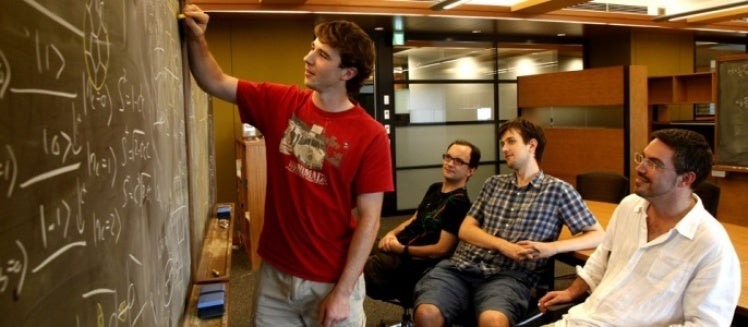The Theory of Quantum Matter (TQM) Unit carries out research into a wide range of problems in condensed matter theory, with a strong emphasis on the novel phases and excitations found in quantum matter.

This work is described in the Annual Reports listed in the menu on the left side of this page.   These provide details of all of the research carried out by the TQM Unit, publications and presentations by Unit members, outreach activity, and seminars given by visitors to TQM in OIST.  Reports are organised by the Japanese financial year, with FY2020 running from April 1st 2020 until March 31st 2021.

Results which appeared since April 1st, 2021, are described below:

### Power-laws in 3-d hard-plate lattice gases.

Fully-packed dimer models on bipartite lattice models are of interest in condensed matter physics as a host to highly-correlated Coulomb liquid phases via an effective field theory in which each fully-packed dimer configuration is mapped to a divergence-free vector field on the links of the links of the lattice. This effective field theory correctly describes the power-law columnar phase in two dimensions and the power-law dipolar correlations seen in three dimensions.

This naturally piques the curiosity on what happens when dimers are generalised to plates, where one plate is a composite object consisting of two parallel dimers with infinite attractive potential. The key question then is whether such a lattice gas displays correlated liquid phases that could be understood by thinking in terms of tensor-valued analogs of the vector fields.

To this end we numerically explore the anisotropic fully-packed model using pocket cluster Monte Carlo algorithm and find that it hosts a crystalline sublattice phase, a columnar phase and a layered phase, wherein the the system separates into bilayers with interlayer and intralayer power-law correlations between plates.

We also explore this model in the presence of vacancies using a transfer matrix based line update Monte Carlo algorithm. In the presence of vacancies, the interlayer power-law correlations in the layered phase decay exponentially. Further increasing the density of vacancies disorders the system.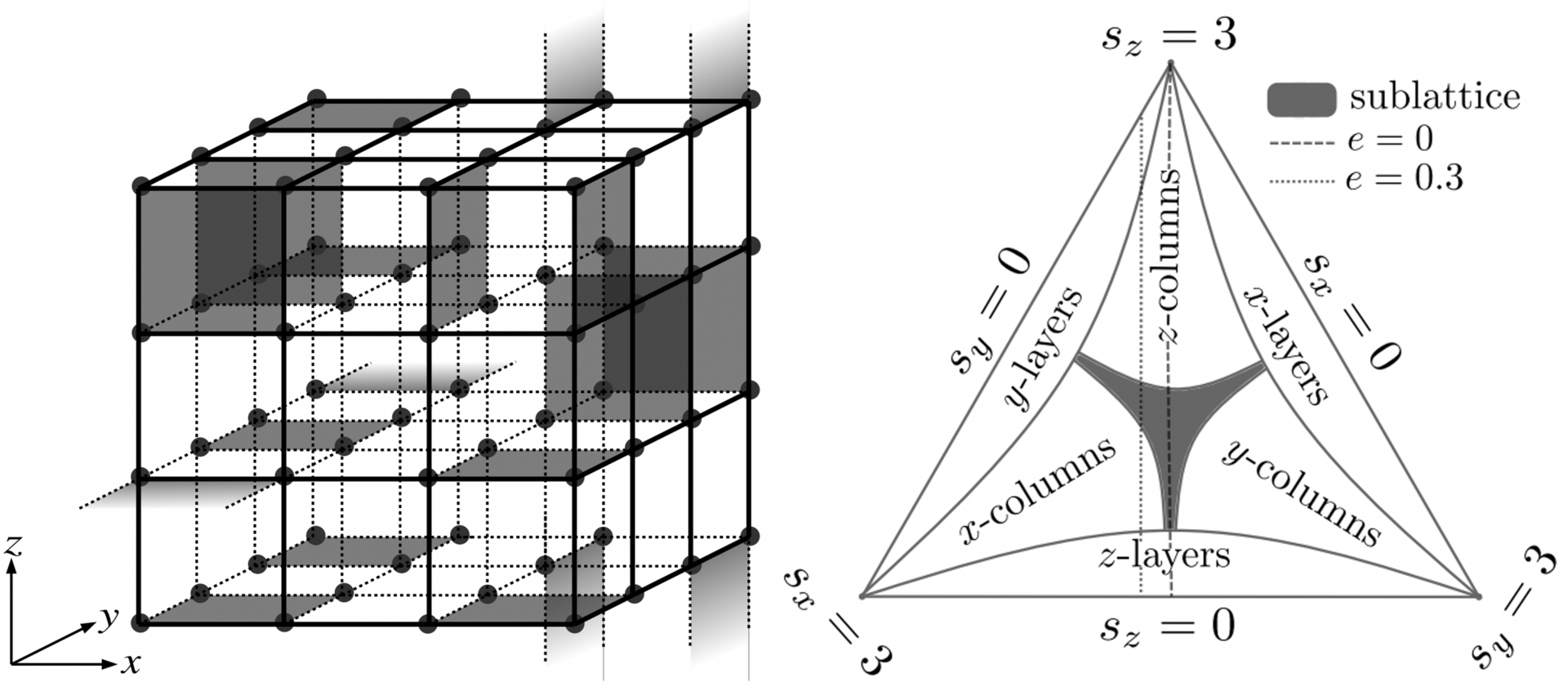Figure on the left shows a configuration of hard plates at full packing on a 4x4 cubic lattice with periodic boundary conditions. Each plate touches exactly four sites of an elementary square plaquette of the cubic lattice, and each site is touched by exactly one such plate in the fully-packed case. Figure on the right shows the phase diagram of the fully-packed anisotropic hard-plate lattice gas on a cubic lattice in the space of anisotropic plate fugacities s . There are three kinds of phases: A sublattice-ordered phase, with lattice translation symmetry broken in all three Cartesian directions, layered phases with lattice translation symmetry broken only along one cartesian direction, and columnar ordered phases with lattice translation symmetry broken along two of the three cartesian directions.

This work is described in the preprint, "Spontaneous layering and power-law order in the three-dimensional fully-packed hard-plate lattice gas" G. Rakala, D. Mandal, S. Biswas, K. Damle, D. Dhar, R. Rajesh, arXiv:2109.02619 and "Phases of the hard-plate lattice gas on a three-dimensional cubic lattice" D. Mandal, G. Rakala, K. Damle, D. Dhar, R. Rajesh", arXiv:2109.02611

### Parafermionic multicriticality in Ising models

The critical properties and associated universality of phase transitions in magnets can be well understood under Wilson’s renormalisation group (RG) framework in terms of the RG flows of the effective Hamiltonian where the nature of the transition is determined by the codimension of the ‘relevant’ coupling constants near the fixed points of the Hamiltonian. Apart from the translational, rotational and global scale invariance present at critical fixed points, a conformal invariance (local scale invariance) was first hypothesised by Polyakov in systems with local interactions.

The fixed points of two-dimensional lattice models with discrete symmetries are described by a class of minimal conformal models with known central charge. Moreover some lattice models show extended superconformal symmetry at criticality between bosons and associated Majorana fermions of the underlying conformal model, making them the first models to confirm the predicted but elusive realization of supersymmetry. In two dimensional planar models with discrete symmetries, a dual parafermionic conformal symmetry was shown to exist. Parafermions are spinful generalisations of Majorana fermions which obey non-Abelian statistics and have been proposed as a robust route to topological quantum computing.

Examples of conformal, superconformal and parafermionic symmetries in condensed matter systems abound and have been of sustained interest. However experimental access to these symmetries is extremely hard due to the presence of two or more ‘relevant’ coupling constants in the vicinity of the associated fixed points of the underlying effective Hamiltonian and thus requiring a fine-tuned control over the parameter space of the physical Hamiltonian.

In this work we study the frustrated antiferromagnetic Ising model on a triangular lattice with further neighbor couplings using worm algorithms. We numerically establish the presence of Z6 parafermionic multicriticality at the pinch-off point of the two-step melting transition. Moreover we show that this multicritical point controls the melting physics in a large experimentally accessible neighbourhood of parameter space.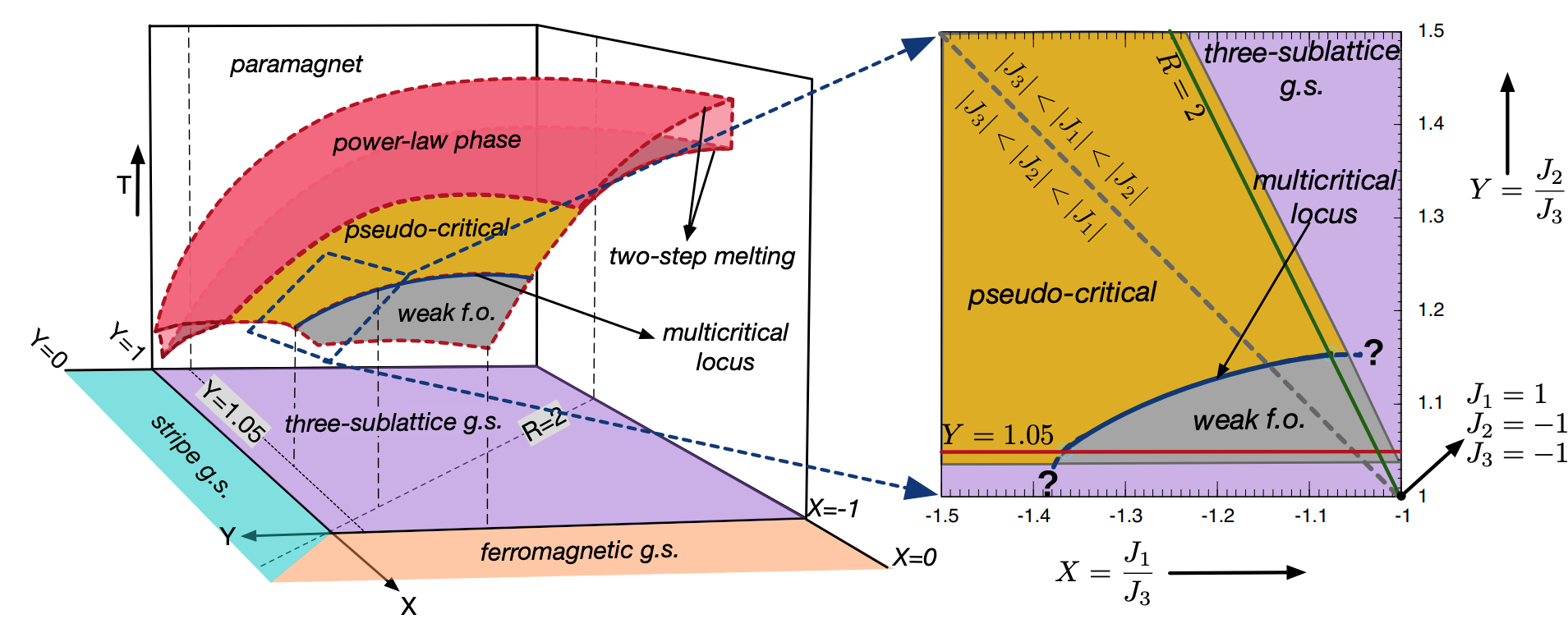The figure shows the melting phase diagram of a triangular lattice antiferromagnet with upto third nearest neighbour interactions. The two-step melting of the three-sublattice order pinches-off into a multicritical locus in the Z6 parafermionic universality class. This multicritical locus controls the critical properties of a large range of experimentally accessible parameter space as shown in the zoomed in region on the right.

This work is described in the preprint, "Melting of three-sublattice order in triangular lattice Ising antiferromagnets: Power-law order, Z6 parafermionic multicriticality, and weakly first order transitions" G. Rakala, N. Desai, S. Shivam, K. Damle, arXiv:2109.03178

### Quantum spin solver near saturation: QS3

Exact diagonalization (ED) method is a traditional and one of the most powerful numerical methods to understand the nature of quantum many-body systems from finite-size cluster calculations. This method enables us to obtain the eigenvectors and eigenvalues of our target Hamiltonian and evaluate all static, dynamical, and thermal quantities without any bias. However, the only disadvantage of the ED method is that the accessible system size is severely limited to small clusters even if we use the modern largest supercomputers. We develop new open-source software -quantum spin solver near saturation: QS3- based on the thick-restart/conventional Lanczos algorithm. This software is powerful for spin-1/2 XXZ-type quantum spin models near fully polarized states, which can be mapped to dilute hardcore Bose systems. All calculations in QS3 are performed in the U(1) and translational symmetry-adapted bases without using the bit representation, and this software can support large-scale finite-size calculations of more than 1000 sites (see Fig. 1). The users can also compute the dynamical spin structure factor by the continuous fractional expansion on the large-scale finite-size cluster (Fig. 2) . The open-source code is available from https://github.com/QS-Cube/ED.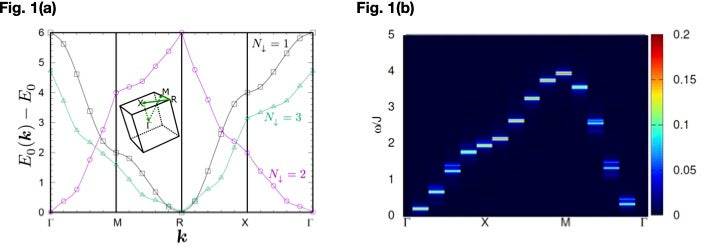(a) The calculation example of the energy dispersions in the S=1/2 simple cubic antiferromagnetic Heisenberg model on the 10*10*10 sites finite-size cluster. (b) The calculation example of the dynamical spin structure factor in the S=1/2 square-lattice antiferromagnetic Heisenberg model on the 10*10 sites finite-size cluster. The details of these computational results are shown in our paper.

This work is described in the preprint, "Quantum spin solver near saturation: QS3" Hiroshi Ueda, Seiji Yunoki and Tokuro Shimokawa, arXiv:2107.00872

### Quantum supremacy, sooner ?

The current generation of quantum computers are exploratory machines, capable of manipulating the information stored in tens of qu-bits, but subject to noise which severely limits the number of operations they can perform, and the accuracy to which they can work.   One of the great challenges of this “noisy, intermediate-scale quantum” (NISQ) era, is to identify algorithms which can exploit the advantages of quantum calculation, while running on a technologically achievable device.

One of the leading contenders is the “quantum approximate optimization algorithm” (QAOA), a recipe for transforming a simple many-qubit wavefunction into one which encodes the solution to a classical optimization problem.    The QAOA works by optimizing the schedule according to which unitary operators are applied to qubits, and is capable of solving problems which would be difficult on a classical computer.   However the accuracy achieved by the QAOA depends heavily on the number of operations performed, with high levels of accuracy requiring more operations than can sensibly be performed on a NISQ device.   Any algorithm which requires less operatorions to achieve the desired accuracy is therefore significant.

In this work, a collaboration of the TQM Unit with researchers from Tsinghua University, The Hong Kong University of Science and Technology, and the University of Wisconsion, Madison, we show how a dramatic speedup in the QAOA can be achieved through the use of an “adaptive bias” (ab-).    In simulations of the solution of the canonical “Maxcut” problem, the ab-QAOA proved to be up to 18 times as fast as the standard QAOA, without any increase in the complexity of the hardware needed to run it.   These results will facilitate the solution of classically hard  optimisation problems using existing quantum computers, and could hasten the day when quantum computers show meaningful advantage over classical computers in real-world problems.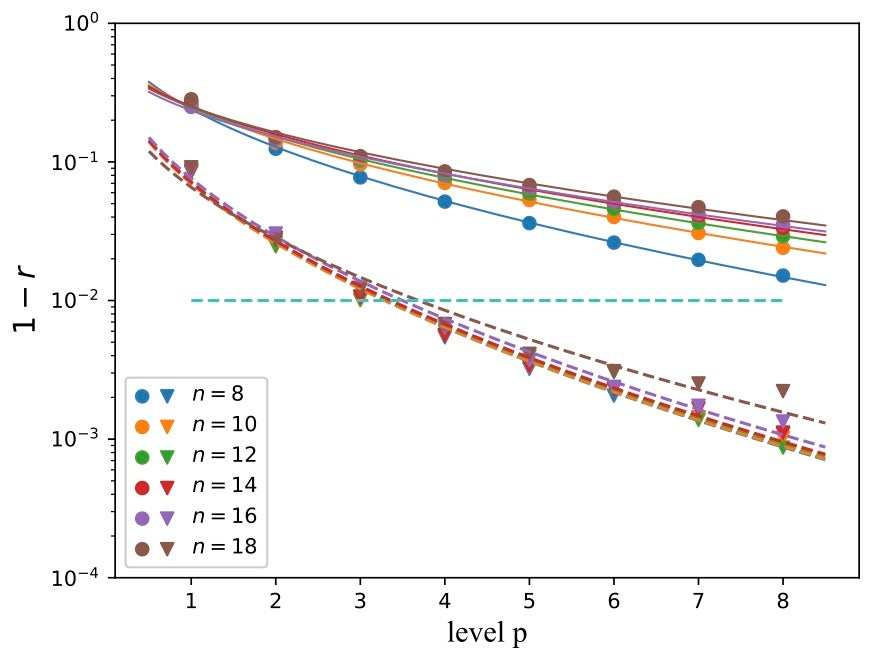Relative error in the solution of the MaxCut problem using the quantum approximate optimization algorithm (QAOA, solid lines), and the QAOA with adaptive bias (ab-QAOA, dashed lines). Results are shown as function of the level of iteration p, for graphs encoded in circuits with n = 8 to 18 qu-bits. Once the adaptive bias is included, a much smaller number of iterations is needed to reach a target accuracy, leading to a dramatic speedup in the algorithm.

This work is described in the preprint “Quantum Approximate Optimization Algorithm with Adaptive Bias Fields”, Yunlong Yu, Chenfeng Cao, Carter Dewey, Xiang-Bin Wang, Nic Shannon, Robert Joynt, arXiv:2105.11946